## Access Control in Networking-

Before you go through this article, make sure that you have gone through the previous article on Access Control.

We have discussed-

• Access Control is a mechanism that controls the access of stations to the transmission link.
• There are various access control methods-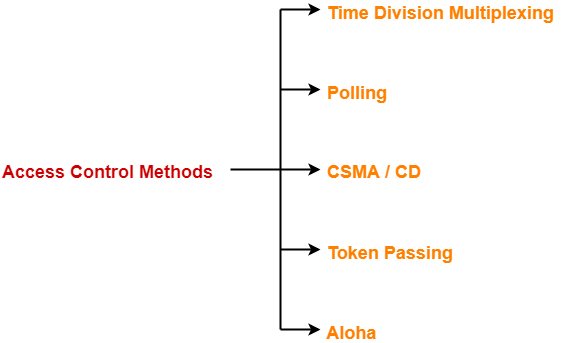## CSMA / CD-

 CSMA / CD stands for Carrier Sense Multiple Access / Collision Detection.

This access control method works as follows-

## Step-01: Sensing the Carrier-

• Any station willing to transmit the data senses the carrier.
• If it finds the carrier free, it starts transmitting its data packet otherwise not.

## How?

• Each station can sense the carrier only at its point of contact with the carrier.
• It is not possible for any station to sense the entire carrier.
• Thus, there is a huge possibility that a station might sense the carrier free even when it is actually not.

## Example-

Consider the following scenario-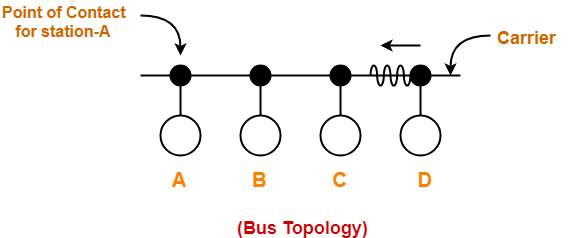At the current instance,

• If station A senses the carrier at its point of contact, then it will find the carrier free.
• But the carrier is actually not free because station D is already transmitting its data.
• If station A starts transmitting its data now, then it might lead to a collision with the data transmitted by station D.

## Step-02: Detecting the Collision-

In CSMA / CD,

• It is the responsibility of the transmitting station to detect the collision.
• For detecting the collision, CSMA / CD implements the following condition.
• This condition is followed by each station-

 Transmission delay >= 2 x Propagation delay

## Meaning-

According to this condition,

• Each station must transmit the data packet of size whose transmission delay is at least twice its propagation delay.
• If the size of data packet is smaller, then collision detection would not be possible.

## Length Of Data Packet-

We know-

• Transmission delay = Length of data packet (L) / Bandwidth (B)
• Propagation delay = Distance between the two stations (D) / Propagation speed (V)

Substituting values in the above condition, we get-

L / B >= 2 x D / V

Thus,

 L >= 2 x B x D / V

### Understanding the Condition To Detect Collision With Example

• Consider at time 10:00 am, station A senses the carrier.
• It finds the carrier free and starts transmitting its data packet to station D.
• Let the propagation delay be 1 hour.

(We are considering station D for the worst case)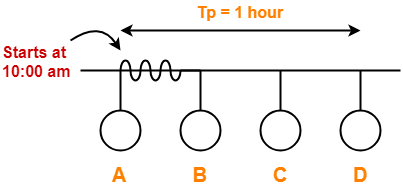• Let us consider the scenario at time 10:59:59:59 when the packet is about to reach the station D.
• At this time, station D senses the carrier.
• It finds the carrier free and starts transmitting its data packet.
• Now, as soon as station D starts transmitting its data packet, a collision occurs with the data packet of station A at time 11:00 am.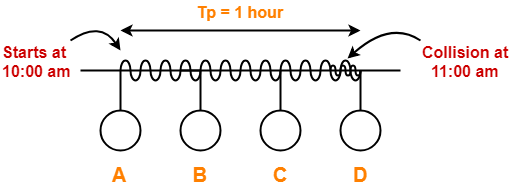• After collision occurs, the collided signal starts travelling in the backward direction.
• The collided signal takes 1 hour to reach the station A after the collision has occurred.
• For station A to detect the collided signal, it must be still transmitting the data.
• So, transmission delay of station A must be >= 1 hour + 1 hour >= 2 hours to detect the collision.
• That is why, for detecting the collision, condition is Tt >= 2Tp.

Two cases are possible-

### Case-01:

If no collided signal comes back during the transmission,

• It indicates that no collision has occurred.
• The data packet is transmitted successfully.

### Case-02:

If the collided signal comes back during the transmission,

• It indicates that the collision has occurred.
• The data packet is not transmitted successfully.
• Step-03 is followed.

## Step-03: Releasing Jam Signal-

• Jam signal is a 48 bit signal.
• It is released by the transmitting stations as soon as they detect a collision.
• It alerts the other stations not to transmit their data immediately after the collision.
• Otherwise, there is a possibility of collision again with the same data packet.
• Ethernet sends the jam signal at a frequency other than the frequency of data signals.
• This ensures that jam signal does not collide with the data signals undergone collision.

## Step-04: Waiting For Back Off Time-

• After the collision, the transmitting station waits for some random amount of time called as back off time.
• After back off time, it tries transmitting the data packet again.
• If again the collision occurs, then station again waits for some random back off time and then tries again.
• The station keeps trying until the back off time reaches its limit.
• After the limit is reached, station aborts the transmission.
• Back off time is calculated using Back Off Algorithm.

## CSMA / CD Flowchart-

The following CSMA / CD flowchart represents the CSMA / CD procedure-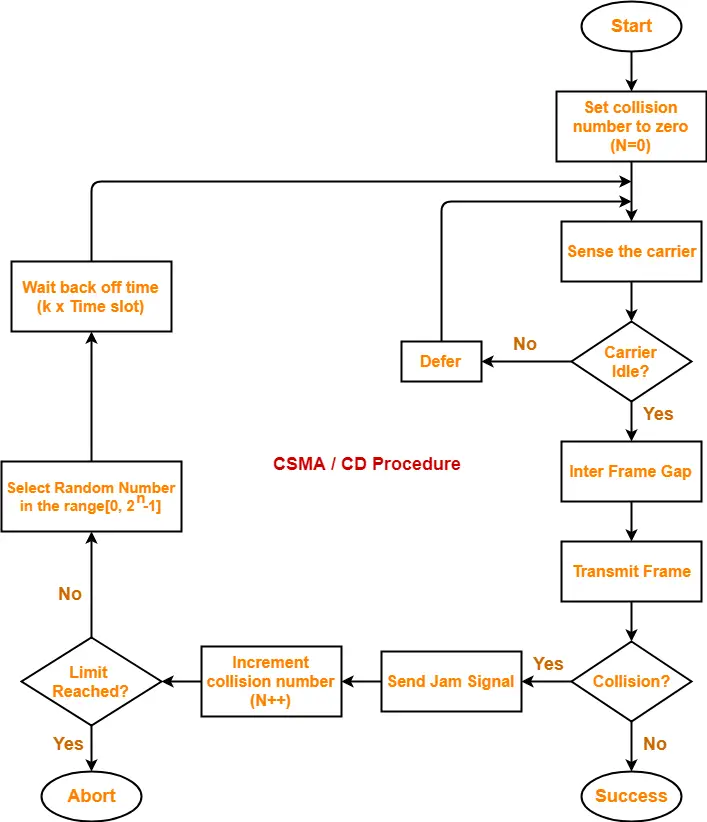## Efficiency-

 Efficiency (η) = Useful Time / Total Time

Before a successful transmission,

• There may occur many number of collisions.
• 2 x Tp time is wasted during each collision.

Thus,

• Useful time = Transmission delay of data packet = Tt
• Useless time = Time wasted during collisions + Propagation delay of data packet = c x 2 x Tp + Tp
• Here, c = Number of contention slots / collision slots.

Thus,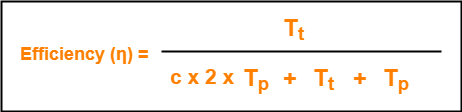Here,

• c is a variable.
• This is because number of collisions that might occur before a successful transmission are variable.

Probabilistic Analysis shows-

 Average number of collisions before a successful transmission = e

Substituting c = e in the above relation, we get-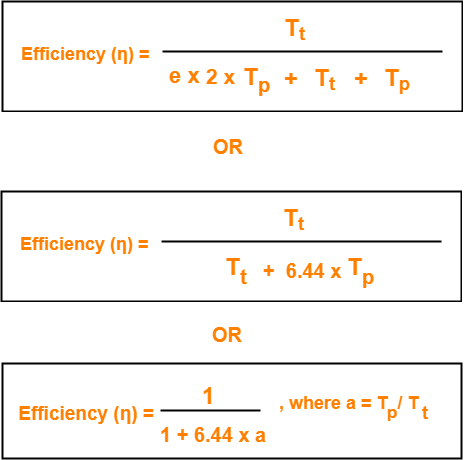## Probabilistic Analysis-

Let us perform the probabilistic analysis to find the average number of collisions before a successful transmission.

Consider-

• Number of stations connected to a CSMA / CD network = n
• Probability of each station to transmit the data = p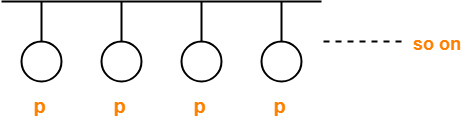Transmission will be successful only when-

• One station transmits the data
• Other (n-1) stations do not transmit the data.

Thus, Probability of successful transmission is given by-Now, let us find the maximum value of Psuccessful transmission.

For maximum value, we put-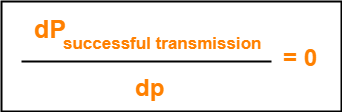On solving,

 At p = 1/n, we get the maximum value of Psuccessful transmission

Thus,

(Psuccessful transmission)max

= nC1 x 1/n x (1 – 1/n)n-1

= n x 1/n x (1 – 1/n)n-1

= (1 – 1/n)n-1

 (Psuccessful transmission)max = (1 – 1/n)n-1

If there are sufficiently large number of stations i.e. n → ∞, then we have-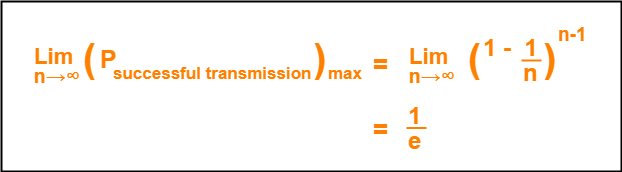Number of times a station must try before successfully transmitting the data packet

= 1 / Pmax         (Using Poisson’s distribution)

= 1 / (1/e)

= e

From here, we conclude-

Average number of collisions that might occur before a successful transmission = e

## Note-01:

• CSMA / CD is used in wired LANs.
• CSMA / CD is standardized in IEEE 802.3

## Note-02:

• CSMA / CD only minimizes the recovery time.
• It does not take any steps to prevent the collision until it has taken place.

## Important Formulas-

• Condition to detect collision: Transmission delay >= 2 x Propagation delay
• Minimum length of data packets in CSMA / CD = 2 x Bandwidth x Distance / Speed
• Efficiency of CSMA / CD = 1 / (1 + 6.44 x a) where a = Tp / Tt
• Probability of successful transmission = nC1 x p x (1-p)n-1
• Average number of collisions before a successful transmission = e

To gain better understanding about CSMA / CD,

Watch this Video Lecture

Next Article- Binary Exponential Back Off Algorithm

Get more notes and other study material of Computer Networks.

Watch video lectures by visiting our YouTube channel LearnVidFun.

## Access Control in Networking-

Before you go through this article, make sure that you have gone through the previous article on Access Control.

We have discussed-

• Access Control is a mechanism that controls the access of stations to the transmission link.
• There are various access control methods-## Polling-

In this access control method,

• A polling is conducted in which all the stations willing to send data participates.
• The polling algorithm chooses one of the stations to send the data.
• The chosen station sends the data to the destination.
• After the chosen station has sent the data, the cycle repeats.

## Example-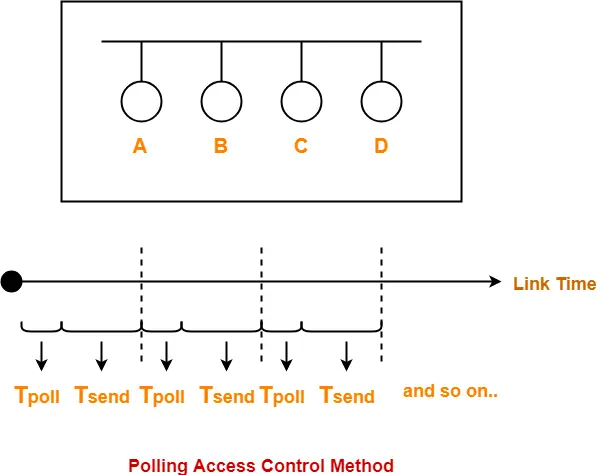Here-

• Tpoll = Time taken for polling
• Tsend = Time taken for sending the data = Transmission delay + Propagation delay = Tt + Tp

## Efficiency-

 Efficiency (η) = Useful Time / Total Time

• Useful time = Transmission delay of data packet = Tt
• Useless time = Time wasted during polling + Propagation delay of data packet = Tpoll + Tp

Thus,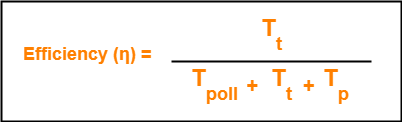• Unlike in Time Division Multiplexing, no slot is ever wasted.
• It leads to maximum efficiency and bandwidth utilization.

• Time is wasted during polling.
• Link sharing is not fair since each station has the equal probability of winning in each round.
• Few stations might starve for sending the data.

## Important Formulas-

• Efficiency (η) = Tt / (Tpoll + Tt + Tp)
• Effective Bandwidth / Bandwidth Utilization / Throughput = Efficiency(η) x Bandwidth
• Maximum Available Effective Bandwidth = Total number of stations x Bandwidth requirement of 1 station

To gain better understanding about Polling Access Control Method,

Watch this Video Lecture

Next Article- CSMA / CD | Access Control Method

Get more notes and other study material of Computer Networks.

Watch video lectures by visiting our YouTube channel LearnVidFun.

## Access Control in Networking-

Before you go through this article, make sure that you have gone through the previous article on Access Control.

We have discussed-

• Access Control is a mechanism that controls the access of stations to the transmission link.
• There are various access control methods-## Time Division Multiplexing-

In Time Division Multiplexing (TDM),

• Time of the link is divided into fixed size intervals called as time slots or time slices.
• Time slots are allocated to the stations in Round Robin manner.
• Each station transmit its data during the time slot allocated to it.
• In case, station does not have any data to send, its time slot goes waste.

## Example-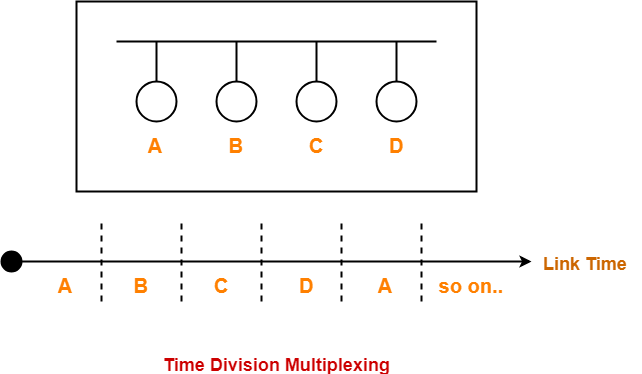## Size Of Time Slots-

The size of each time slot is kept such that each station gets sufficient time for the following tasks-

• To put its data packet on to the transmission link
• Last bit of the packet is able to get out of the transmission link

Thus,

 Size of each time slot = Tt + Tp

where-

• Tt = Transmission delay
• Tp = Propagation delay

## NOTE-

To keep the size of time slots constant,

• We have assumed that all the stations want to send the packets of same size.
• This keeps Tt constant for all the stations.
• We have considered the worst case when both the stations are present at the two extreme ends.
• This ensures Tp will be maximum and all the stations will get sufficient time to propagate their data.

## Efficiency-

 Efficiency (η) = Useful Time / Total Time

• Useful time = Transmission delay of data packet = Tt
• Useless time = Propagation delay of data packet = Tp

Thus,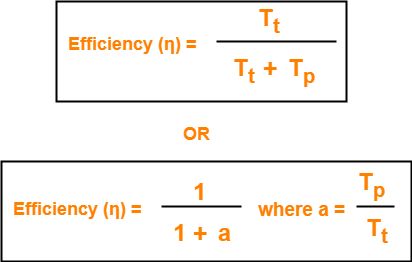## Important Formulas-

• Size of each time slot in Time Division Multiplexing = Tt + Tp
• Efficiency (η) = 1 / (1+a) where a = Tp / Tt
• Effective Bandwidth / Bandwidth Utilization / Throughput = Efficiency(η) x Bandwidth
• Maximum Available Effective Bandwidth = Total number of stations x Bandwidth requirement of 1 station

• If any station does not have the data to send during its time slot, then its time slot goes waste.
• This reduces the efficiency.
• This time slot could have been allotted to some other station willing to send data.

## Problem-

If transmission delay and propagation delay of a packet in Time Division Multiplexing is 1 msec each at 4 Mbps bandwidth, then-

1. Find the efficiency.
2. Find the effective bandwidth.
3. How many maximum stations can be connected to the network if each station requires 2 Kbps bandwidth?

## Solution-

Given-

• Transmission delay (Tt) = 1msec
• Propagation delay (Tp) = 1msec
• Bandwidth = 4 Mbps

## Part-01:

For a TDM Network,

 Efficiency (η) = 1 / 1+a where a = Tp / Tt

### Calculating Value Of ‘a’-

a = Tp / Tt

a = 1 msec / 1 msec

a = 1

Efficiency (η)

= 1 / (1+a)

= 1 / (1 + 1)

= 1 / 2

= 0.5

= 50%

## Part-02:

We know-

 Effective Bandwidth = Efficiency (η) x Bandwidth

Thus,

Effective Bandwidth

= 0.5 x 4 Mbps

= 2 Mbps

## Part-03:

We know-

 Maximum Effective Bandwidth= Total number of stations x Bandwidth requirement of 1 station

Let the total number of stations that can be connected be N.

Then, we have-

2 Mbps = N x 2 Kbps

N = 1000

Thus, maximum 1000 stations can be connected.

To gain better understanding about Time Division Multiplexing (TDM),

Watch this Video Lecture

Next Article- Polling | Access Control Method

Get more notes and other study material of Computer Networks.

Watch video lectures by visiting our YouTube channel LearnVidFun.

## Access Control in Networking | Methods

In computer networking,

• Communication links enable the stations to communicate with each other.
• Stations may communicate using the following types of links-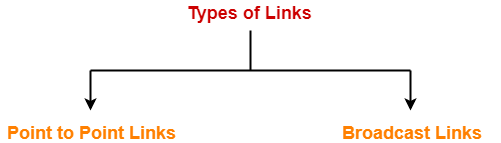## 1. Point to Point Link-

• Point to Point link is a dedicated link that exists between the two stations.
• The entire capacity of the link is used for transmission between the two connected stations only.
• Depending upon the Type Of Channel, the data flow takes place between the stations.

## Example-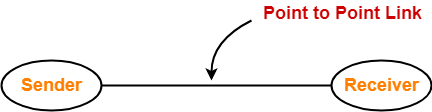• The capacity of the link is shared among the connected stations for transmission.

## Example-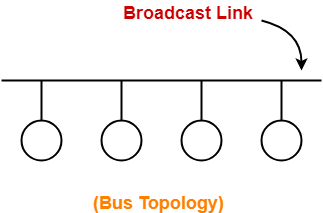## Access Control-

 Access Control is a mechanism that controls the access of stations to the transmission link.

• This is because the link is shared among several stations.

## Need of Access Control-

 To prevent the occurrence of collision or if the collision occurs, to deal with it.

Consider a situation where-

• Multiple stations place their data packets on the link and starts transmitting simultaneously.
• Such a situation gives rise to a collision among the data packets.
• Collision of data packets causes the data to get corrupt.

## Example-

Consider the following scenario-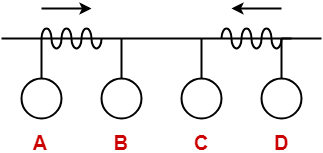Here,

• Two stations A and D starts transmitting their data packets simultaneously.
• This situation gives rise to a collision between the data packets transmitted by them.
• Thus, to prevent the collision or to deal with it, access control is needed.

## Access Control Methods-

 Access control methods are the methods used for providing access control.

• They prevent the collision or deal with it and ensures smooth flow of traffic on the network.
• They are implemented at the data link layer of the OSI reference model.

Various access control methods used are-We will discuss all these methods one by one in detail.

To gain better understanding about Access Control,

Watch this Video Lecture

Next Article- Time Division Multiplexing (TDM)

Get more notes and other study material of Computer Networks.

Watch video lectures by visiting our YouTube channel LearnVidFun.